NEET  >  Test: NEET Previous Year Questions: Work, Energy & Power - 2

# Test: NEET Previous Year Questions: Work, Energy & Power - 2

Test Description

## 9 Questions MCQ Test Physics Class 11 | Test: NEET Previous Year Questions: Work, Energy & Power - 2

Test: NEET Previous Year Questions: Work, Energy & Power - 2 for NEET 2023 is part of Physics Class 11 preparation. The Test: NEET Previous Year Questions: Work, Energy & Power - 2 questions and answers have been prepared according to the NEET exam syllabus.The Test: NEET Previous Year Questions: Work, Energy & Power - 2 MCQs are made for NEET 2023 Exam. Find important definitions, questions, notes, meanings, examples, exercises, MCQs and online tests for Test: NEET Previous Year Questions: Work, Energy & Power - 2 below.
Solutions of Test: NEET Previous Year Questions: Work, Energy & Power - 2 questions in English are available as part of our Physics Class 11 for NEET & Test: NEET Previous Year Questions: Work, Energy & Power - 2 solutions in Hindi for Physics Class 11 course. Download more important topics, notes, lectures and mock test series for NEET Exam by signing up for free. Attempt Test: NEET Previous Year Questions: Work, Energy & Power - 2 | 9 questions in 10 minutes | Mock test for NEET preparation | Free important questions MCQ to study Physics Class 11 for NEET Exam | Download free PDF with solutions
 1 Crore+ students have signed up on EduRev. Have you?
Test: NEET Previous Year Questions: Work, Energy & Power - 2 - Question 1

### An ideal spring with spring-constant k is hung from the ceiling and a block of mass M is attached to its lower end. The mass is released with the spring initially unstretched. Then the maximum extension in the spring is

Detailed Solution for Test: NEET Previous Year Questions: Work, Energy & Power - 2 - Question 1

Once the mass is released, the spring would extend Mg/k length where it comes to an equilibrium, but by then the mass would have gained some speed due to which the spring will be stretched Mg/k length more and then start a damped oscillation. Thus maximum extension by spring is 2Mg/k length.

Test: NEET Previous Year Questions: Work, Energy & Power - 2 - Question 2

### A spherical ball of mass m is kept at the highest point in the space between two fixed, concentric spheres A and B (see figure.) The smaller sphere A has a radius R and the space between the two spheres has a width d. The ball has a diameter very slightly less than d. All surfaces are frictionless. The ball is given a gentle push (towards the right in the figure). The angle made by the radius vector of the ball with the upward vertical is denoted by q (shown in the figure) [JEE-2002] (a) Express the total normal reaction force exerted by the spheres on the ball as a function of angle q.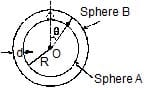(b) Let NA and NB denote the magnitudes of the normal reaction force on the ball exerted by the spheres A and B, respectively. Sketch the variations of NA and NB as functions of cosq in the range 0 £ q £ p by drawing two separate graphs in your answer book, taking cosq on the horizontal axes.

Test: NEET Previous Year Questions: Work, Energy & Power - 2 - Question 3

### In a region of only gravitational field of mass `M' a particle is shifted from A to B via three different paths in the figure. The work done in different paths are W1, W2, W3 respectively then [JEE(Scr.)-2003]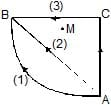Detailed Solution for Test: NEET Previous Year Questions: Work, Energy & Power - 2 - Question 3

Since gravitational force is conservative in nature, so, only initial and final position matter.

Test: NEET Previous Year Questions: Work, Energy & Power - 2 - Question 4

A particle is placed at the origin and a force F = kx is acting on it (where k is a positive constant). If U(0) = 0, the graph of U(x) versus x will be (where U is the potential energy function)

[JEE(Scr.)-2004]

Detailed Solution for Test: NEET Previous Year Questions: Work, Energy & Power - 2 - Question 4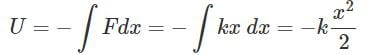This is the equation of parabola symmetric to U axis in negative direction.

Test: NEET Previous Year Questions: Work, Energy & Power - 2 - Question 5

A bob of mass M is suspended by a massless string of length L. The horizontal velocity V at position A is just sufficient to make it reach the point B. The angle q at which the speed of the bob is half of that at A, satisfies

Detailed Solution for Test: NEET Previous Year Questions: Work, Energy & Power - 2 - Question 5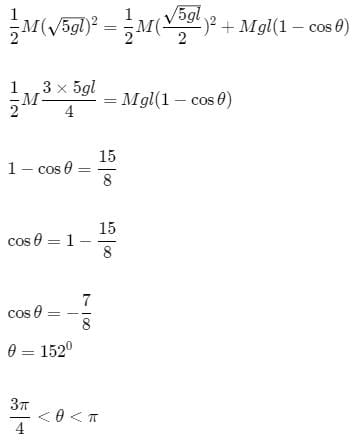Test: NEET Previous Year Questions: Work, Energy & Power - 2 - Question 6

A light inextensible string that goes over a smooth fixed pulley as shown in the figure connects two blocks of masses 0.36 kg and 0.72 kg. Taking g = 10 m/s2, find the work done (in joules) by the string on the block of mass 0.36 kg during the first second after the system is released from rest.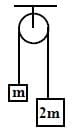Detailed Solution for Test: NEET Previous Year Questions: Work, Energy & Power - 2 - Question 6

2mg − T = (2m)a
T − mg = ma
⇒ a = g/3
T = 4mg/3
w = T × s
= T × 1/2at2
= 4/3mg * 1/2 * g/3 * 12
= 200/9 * 0.36
= 8 Joules

Test: NEET Previous Year Questions: Work, Energy & Power - 2 - Question 7

A ball of mass (m) 0.5 kg is attached to the end of a string having length (L) 0.5 m. The ball is rotated on a horizontal circular path about vertical axis. The maximum tension that the string can bear is 324 N. the maximum possible value of angular velocity of ball (in radian/s) is

[jee-2011]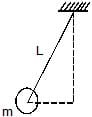Detailed Solution for Test: NEET Previous Year Questions: Work, Energy & Power - 2 - Question 7

Forum id- 1557839
We have,
Tsinθ=mrω2
But,
sinθ=Lr​
∴ T=mLω2
∴ 324=0.5×0.5×ω2

*Answer can only contain numeric values
Test: NEET Previous Year Questions: Work, Energy & Power - 2 - Question 8

A block of mass 0.18 kg is attached to a spring of force-constant 2 N/m. The coefficient of friction between the block and the floor is 0.1. Initially the block is at rest and the spring is un-stretched. An impulse is given to the block as shown in the figure. The block slides a distance of 0.06 m and comes to rest for the first time. The initial velocity of the block in m/s is V = N/10. Then N is

[JEE-2011]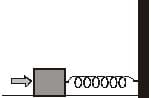Test: NEET Previous Year Questions: Work, Energy & Power - 2 - Question 9

Two identical discs of same radius R are rotating about their axes in opposite directions with the same constant angular speed w. The discs are in the same horizontal plane At time t = 0, the points P and Q are facing each other as shown in the figure. The relative speed between the two points P and Q is nr.In one time period (T) of rotation of the discs, nr as a function of time is best represented by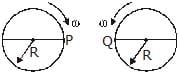[JEE-2012]

Detailed Solution for Test: NEET Previous Year Questions: Work, Energy & Power - 2 - Question 9

Draw tangents to the circles at P and Q and at the top and bottom of the circles and mark the direction of motion on each.
As, both speeds are equal and at P and Q, the direction of motion is the same, relative speed is 0.

At the top and bottom of the path, the direction of motion of each of them is opposite to that of the other. Thus, relative speed increases.
At the diametrically opposite positions to P and Q, again the motion is in the same direction and the speeds are equal, so, relative speed is 0.
Thus, the relative speed increases from 0 to a maximum value as the discs rotate from P and Q to the bottom of the path , then it decreases to 0 as they rotate from the bottom to the diametrically opposite positions to P and Q , and again increases as they rotate to the top and finally becomes 0 as they come back again to their original position.

## Physics Class 11

127 videos|464 docs|210 tests
 Use Code STAYHOME200 and get INR 200 additional OFF Use Coupon Code
Information about Test: NEET Previous Year Questions: Work, Energy & Power - 2 Page
In this test you can find the Exam questions for Test: NEET Previous Year Questions: Work, Energy & Power - 2 solved & explained in the simplest way possible. Besides giving Questions and answers for Test: NEET Previous Year Questions: Work, Energy & Power - 2, EduRev gives you an ample number of Online tests for practice

## Physics Class 11

127 videos|464 docs|210 tests

### How to Prepare for NEET

Read our guide to prepare for NEET which is created by Toppers & the best Teachers